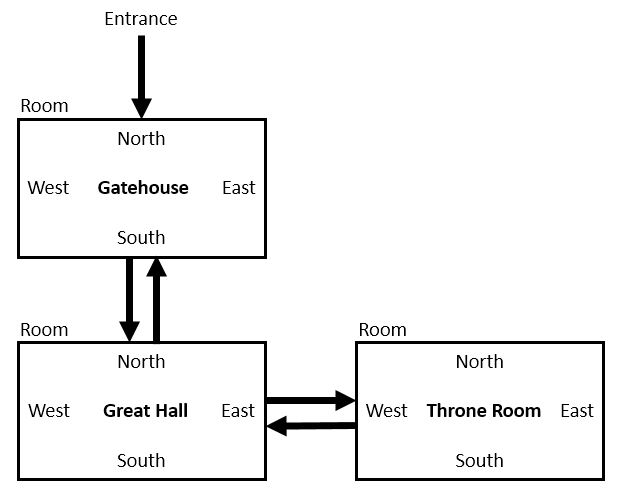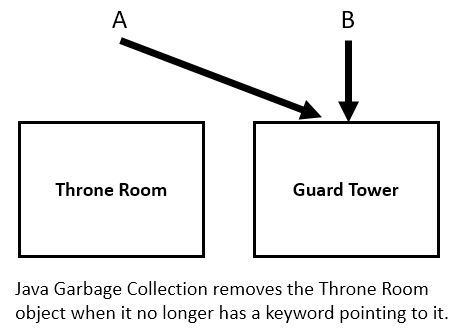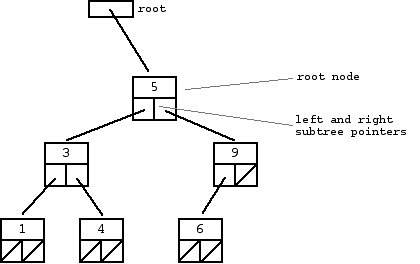Java Chapter 15 - Linked Lists and Trees

15.1 Java References

Object keywords are references which is an address that tells where the object's class variables and objects are stored.  Unlike C++, Java does not have pointers which allows variables to store memory addresses and can be changed to point to arbitrary addresses.  We can, however, use these references in some ways like pointers to create various data structures.  Look at the code below.  Only one object is being created since there's only one new statement.  Both A and B reference the same object.  Therefore, when you increment B.Apples, A.Apples shows the same value.

 reference.java ```public class reference { public static void main(String[] args) { Stuff A = new Stuff(); A.Apples = 7; Stuff B = A; // B refererences or points to the same object as A B.Apples++; System.out.println(A.Apples); } } class Stuff { int Apples; }``` Output 8

The code below shows how to use objects and references to configure the rooms of a castle that you can be travel through.  Entrance is the keyword that points to the first object - Gatehouse. T is a temporary keyword used to create the Great Hall and Throne Room objects.  Each Room has a pointer to another Room - North, South, East, and West.  These can be connected

 castle.java```public class castle { public static void main(String[] args) { Room Entrance = new Room("Gatehouse"); Room T = new Room("Great Hall"); T.North = Entrance; // Great Hall North points to Gatehouse Entrance.South = T; // Gatehouse South points to Great Hall T = new Room("Throne Room"); T.West = Entrance.South; // Throne Room West points to Great Hall Entrance.South.East = T; // Great Hall East points to Throne Room System.out.println(Entrance.South.East.RoomName); System.out.println(Entrance.South.East.West.North.RoomName); } } class Room { String RoomName; Room North, South, East, West; Room (String TheRoomName) { RoomName = TheRoomName; } }``` Output Throne Room Gatehouse

 Java Garbage Collection Java has built in memory management which frees the programmer from the task of reclaiming memory of unused objects.  If you create an object and reassign the keyword that points to it, Java's garbage collection removes the object from memory.  For example: Room A = new Room ("Throne Room"); Room B = new Room ("Guard Tower"); A = B;A linked list is a data structure made of nodes where each node has a pointer to the next.  The last node's pointer is null.On average, the time it takes to locate an item in a linked list is O(n).

In Java, you can implement a linked list using Node classes.  Notice that Next is a pointer to the next Node.

class Node
{
int Data;
Node Next;

Node()
{
Next = null;
}
}

To insert a new node at the head of the list, you could do the following:

Node T = new Node();
T.Data = NewData;
// first Node of the list
{
}
else
// insert node between Head and first Node
{
}

15.3 Binary Tree

A binary tree is a data structure made of nodes, where each node contains a left pointer, a right pointer, and a data element.  If the tree is empty, then the root pointer is null.

A recursive definition of this data structure is:  a binary tree is either empty (null pointer), or a single node where the left and right pointers each point to another binary tree.

A binary search tree (BST) is an ordered binary tree where all the elements in the left search tree are <= the node and all the elements in the right search tree are >= node.On average, a BST can be searched in O(log n).

In Java, you can implement a BST using Node classes.  Notice that Left and Right are pointers to another Node.

class Node
{
int Data;
Node Left, Right;

Node()
{
Left = null;
Right = null;
}
}

Implementing a binary tree of integers using the Node class could use have these recursive methods:

static boolean Locate(Node TheNode, int TheData)
{
if (TheNode == null)
return false;
if (TheNode.Data == TheData)
return true;
else if (TheNode.Data > TheData) // traverse tree to the left
return Locate(TheNode.Left,TheData);
else if (TheNode.Data < TheData) // traverse tree to the right
return Locate(TheNode.Right,TheData);
else if (TheNode.Data > TheData && TheNode.Left == null)
return false;
else if (TheNode.Data < TheData && TheNode.Right == null)
return false;
return false; // Java needs a default return
}

static void Insert(Node TheNode, int NewData)
{
if (TheNode.Data > NewData && TheNode.Left == null)
// Left is null - add new node on left
{
Node T = new Node();
T.Data = NewData;
TheNode.Left = T;
}
else if (TheNode.Data < NewData && TheNode.Right == null)
// Right is null - add new node on right
{
Node T = new Node();
T.Data = NewData;
TheNode.Right = T;
}
else if (TheNode.Data > NewData && TheNode.Left != null)
// Left isn't null - recurse to left
Insert(TheNode.Left, NewData);
else if (TheNode.Data < NewData && TheNode.Right != null)
// Right isn't null - recurse to right
Insert(TheNode.Right, NewData);
}